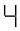# vs.eyeandcontacts.com

## Chapter 12 Algebraic Expressions Exercise 12.4

Question 1: Observe the patterns of digits made from line segments of equal length. You will find such segmented digits on the display of electronic watches or calculators.

If the number of digits formed is taken to be n, the number of segments required to form n digits is given by the algebraic expression appearing on the right of each pattern. How many segments are required to form 5, 10, 100 digits of the kind

(a)is (5n + 1)
The number of segments required to form 5 digits = ((5 × 5) + 1)
= (25 + 1)
= 26
The number of segments required to form 10 digits = ((5 × 10) + 1)
= (50 + 1)
= 51
The number of segments required to form 100 digits = ((5 × 100) + 1)
= (500 + 1)
= 501

(b)is (3n + 1)
The number of segments required to form 5 digits = ((3 × 5) + 1)
= (15 + 1)
= 16
The number of segments required to form 10 digits = ((3 × 10) + 1)
= (30 + 1)
= 31
The number of segments required to form 100 digits = ((3 × 100) + 1)
= (300 + 1)
= 301

(c)is (5n + 2)
The number of segments required to form 5 digits = ((5 × 5) + 2)
= (25 + 2)
= 27
The number of segments required to form 10 digits = ((5 × 10) + 2)
= (50 + 2)
= 52
The number of segments required to form 100 digits = ((5 × 100) + 1)
= (500 + 2)
= 502

Question 2: Use the given algebraic expression to complete the table of number patterns.
(i) 2n – 1
Then, 100th term =?
n = 100
= (2 × 100) – 1
= 200 – 1
= 199

(ii) 3n + 2
5th term =?
n = 5
= (3 × 5) + 2
= 15 + 2
= 17
10th term =?
n = 10
= (3 × 10) + 2
= 30 + 2
= 32
100th term =?
n = 100
= (3 × 100) + 2
= 300 + 2
= 302

(iii) 4n + 1
5th term =?
n = 5
= (4 × 5) + 1
= 20 + 1
= 21
10th term =?
n = 10
= (4 × 10) + 1
= 40 + 1
= 41
100th term =?
n = 100
= (4 × 100) + 1
= 400 + 1
= 401

(iv) 7n + 20
5th term =?
n = 5
= (7 × 5) + 20
= 35 + 20
= 55
10th term =?
n = 10
= (7 × 10) + 20
= 70 + 20
= 90
100th term =?
n = 100
= (7 × 100) + 20
= 700 + 20
= 720

(v) n2 + 1
5th term =?
n = 5
= (52) + 1
= 25+ 1
= 26
10th term =?
n = 10
= (102) + 1
= 100 + 1
= 101

 S. No. Expression Terms 1st 2nd 3rd 4th 5th … 10th … 100th … (i) 2n – 1 1 3 5 7 9 – 19 – 199 – (ii) 3n + 2 5 8 11 14 17 – 32 – 302 – (iii) 4n + 1 5 9 13 17 21 – 41 – 401 – (iv) 7n + 20 27 34 41 48 55 – 90 – 720 – (v) n2 + 1 2 5 10 17 26 – 101 – 10001 –